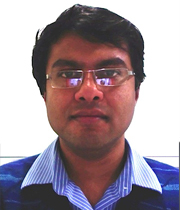# Integral Transforms And Their Applications

By Prof. Sarthok Sircar   |   IIIT Delhi
Learners enrolled: 606
The course is designed as an introduction to the theory and applications of integral transforms to problems in linear differential equations, to boundary and initial value problems in partial differential equations and continuum mechanics. Many new applications in applied mathematics, physics, chemistry, biology and engineering are included. This course will serve as a reference for advanced study and research in this subject as well as for its applications in the fields of signal processing, informatics and communications, neuroscience, fluid mechanics, quantum mechanics, computer assisted tomography (CAT). The course is open to all MTech, PhD students, some final year advanced undergraduate and honors students.

INTENDED AUDIENCE: Any interested learners
PREREQUISITES: 1) Ordinary Differential Equations (ODEs) ,2) Complex variables (optional)
INDUSTRY SUPPORT: (1) Signal Processing and Communications, (2) Data Science, (3) Computational Fluid Dynamics, (4) Software Development
Summary
 Course Status : Completed Course Type : Elective Duration : 12 weeks Start Date : 26 Jul 2021 End Date : 15 Oct 2021 Exam Date : 24 Oct 2021 IST Enrollment Ends : 09 Aug 2021 Category : Mathematics Credit Points : 3 Level : Undergraduate/Postgraduate

### Course layout

Week 1:
Basic concepts of integral transforms.
Fourier transforms: Introduction, basic properties, applications to solutions of Ordinary Differential Equations (ODE),
Partial Differential Equations (PDE).
Week 2:
Applications of Fourier Transforms to solutions of ODEs, PDEs and Integral Equations,
evaluation of definite integrals.
Laplace transforms: Introduction, existence criteria
Week 3:
Laplace transforms: Convolution, differentiation, integration, inverse transform,
Tauberian Theorems, Watson’s Lemma, solutions to ODE, PDE including Initial Value Problems (IVP) and Boundary Value Problems (BVP).
Week 4:
Applications of joint Fourier-Laplace transform, definite integrals, summation of infinite series, transfer functions, impulse response function of linear systems.
Week 5:
Hankel Transforms: Introduction,
properties and applications to PDE Mellin transforms: Introduction, properties, applications;
Generalized Mellin transforms.
Week 6:
Hilbert Transforms: Introduction, definition, basic properties, Hilbert transforms in complex plane, applications;
asymptotic expansions of 1-sided Hilbert transforms.
Week 7:
Stieltjes Transform: definition, properties, applications, inversion theorems, properties of generalized Stieltjes transform.
Legendre transforms: Intro, definition, properties, applications.
Week 8:
Z Transforms: Introduction, definition, properties; dynamic linear system and impulse response, inverse Z transforms,
summation of infinite series, applications to finite differential equations
Week 9: Radon transforms: Introduction, properties, derivatives, convolution theorem, applications, inverse radon transform.
Week 10: Fractional Calculus and its applications: Intro, fractional derivatives, integrals, Laplace transform of fractional integrals and derivatives.
Week 11:
Integral transforms in fractional equations: fractional ODE, integral equations,
IVP for fractional Differential Equations (DE), fractional PDE, green’s function for fractional DE.
Week 12: Wavelet Transform: Discussion on continuous and discrete, Haar, Shannon and Daubechie Wavelets.

### Books and references

1) Advanced Topics in Applied Mathematics for Engg. & physical Science: Sudhakar Nair
2) Introduction to Applied Mathematics, Gilbert Strang
3) Fractional Calculus Theory and Applications of Differentiation and Integration to Arbitrary Order: J. Spanier and K. B. Oldham
4) Handbook of Mathematical Functions: M. Abramowitz & I. Stegun

### Instructor bio### Prof. Sarthok Sircar

IIIT Delhi
I am currently an Assistant Professor in the Department of Mathematics at Indraprastha Institute for Information Technology, Delhi. My prior academic appointments include (a) Lectureship in the Division of Mathematical Sciences, University of Adelaide, Australia, (b) Research Associate in Division of Applied Mathematics, University of Colorado, Boulder, (c) Research Fellow in Biomathematics in the University of Utah, (d) Visiting Scholar in the Center for Nanophase Material Science at Oak Ridge National Laboratory, and (e) Research Scientist in Corning Inc. at Ithaca, NY. My main mathematical interests are in the development and analysis of nonlinear hyperbolic and elliptic partial differential equations, with applications which lie at the interface of applied mathematics and biology. I am particularly interested in solving problems involving soft matter and fluid flow using asymptotic and perturbation methods, numerical approximation and statistical mechanics.

### Course certificate

The course is free to enroll and learn from. But if you want a certificate, you have to register and write the proctored exam conducted by us in person at any of the designated exam centres.
The exam is optional for a fee of Rs 1000/- (Rupees one thousand only).
Date and Time of Exams: 24 October 2021 Morning session 9am to 12 noon; Afternoon Session 2pm to 5pm.
Registration url: Announcements will be made when the registration form is open for registrations.
The online registration form has to be filled and the certification exam fee needs to be paid. More details will be made available when the exam registration form is published. If there are any changes, it will be mentioned then.
Please check the form for more details on the cities where the exams will be held, the conditions you agree to when you fill the form etc.

CRITERIA TO GET A CERTIFICATE

Average assignment score = 25% of average of best 8 assignments out of the total 12 assignments given in the course.
Exam score = 75% of the proctored certification exam score out of 100

Final score = Average assignment score + Exam score

YOU WILL BE ELIGIBLE FOR A CERTIFICATE ONLY IF AVERAGE ASSIGNMENT SCORE >=10/25 AND EXAM SCORE >= 30/75. If one of the 2 criteria is not met, you will not get the certificate even if the Final score >= 40/100.

Certificate will have your name, photograph and the score in the final exam with the breakup.It will have the logos of NPTEL and IIIT Madras.It will be e-verifiable at nptel.ac.in/noc.

Only the e-certificate will be made available. Hard copies will not be dispatched.

Once again, thanks for your interest in our online courses and certification. Happy learning.

- NPTEL team